Concepts

Class 10
Chapter 4 Class 10 - Carbon and its Compounds

# Alkanes

## What are Alkanes:

These are the hydrocarbons in which carbon atoms are linked to each other by a single bond.

### Example: Methane

• Formula of Methane is CH 4
• There is only one Carbon Atom.
• The 1 Carbon atom has 4 Valence electrons .
• It shares these 4 Valence electrons (1 electron each) with 4 Hydrogen Atoms.

A methane molecule has 1 carbon atom and 4 hydrogen atoms .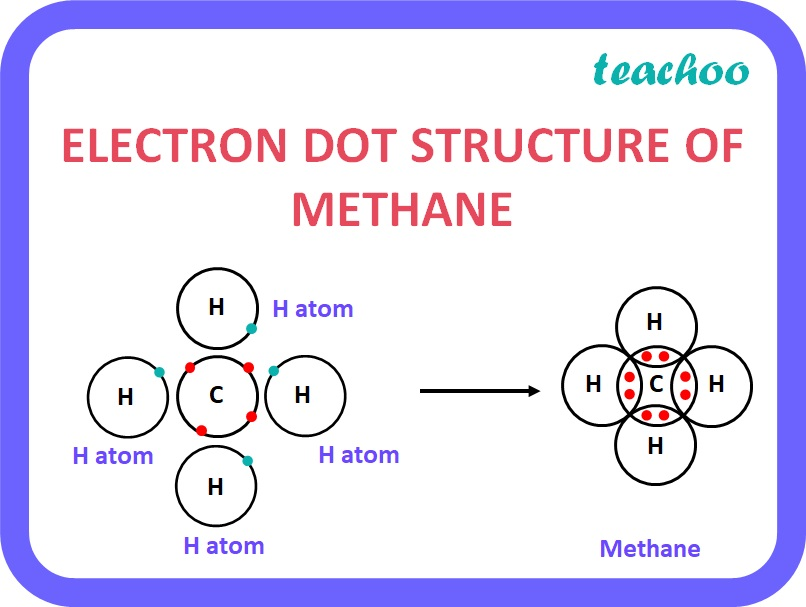## General formula for Alkanes:

It Is C n H 2n+2

• Where n is the number of carbon atoms.

 Number of Carbon Atoms (n) n=1 n=2 n=3 Name of Alkane Methane Ethane Propane Structure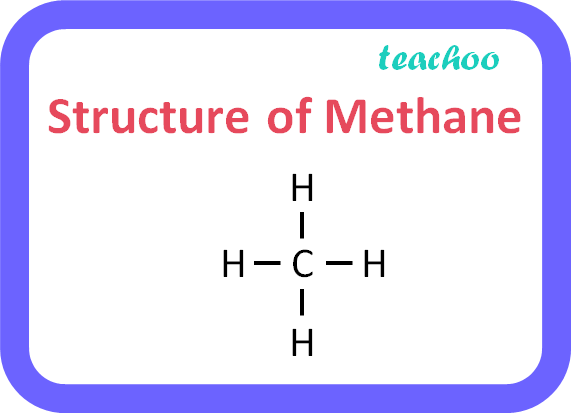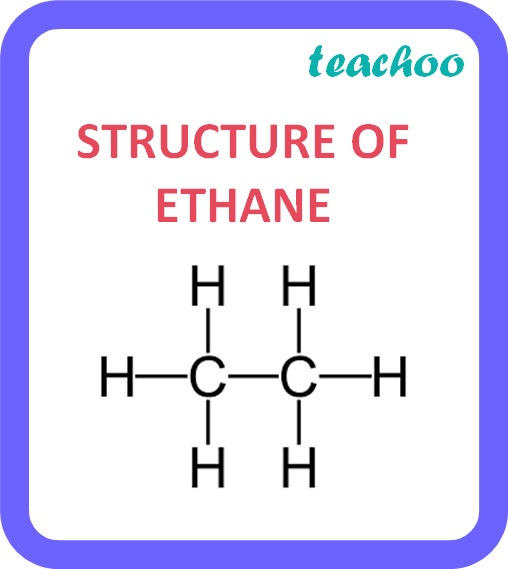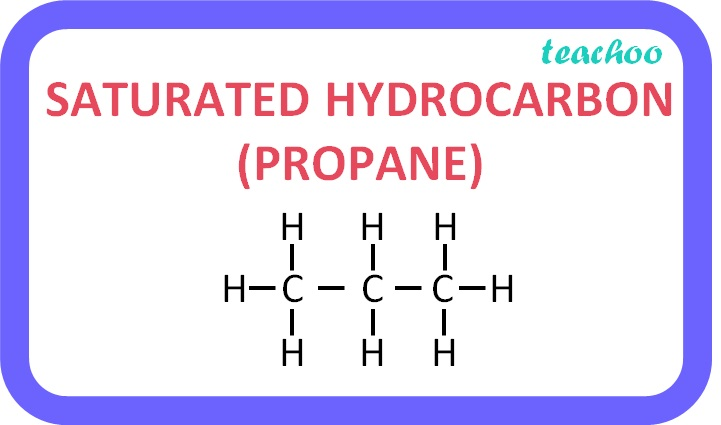### Propane

• In Propane, there are 3 carbon atoms
• The general formula for alkanes is:

C n H 2n+2

• Putting n=3,

C 3 H 3*2+2

C 3 H 6+2

C 3 H 8

Hence, the formula for propane is, C 3 H 8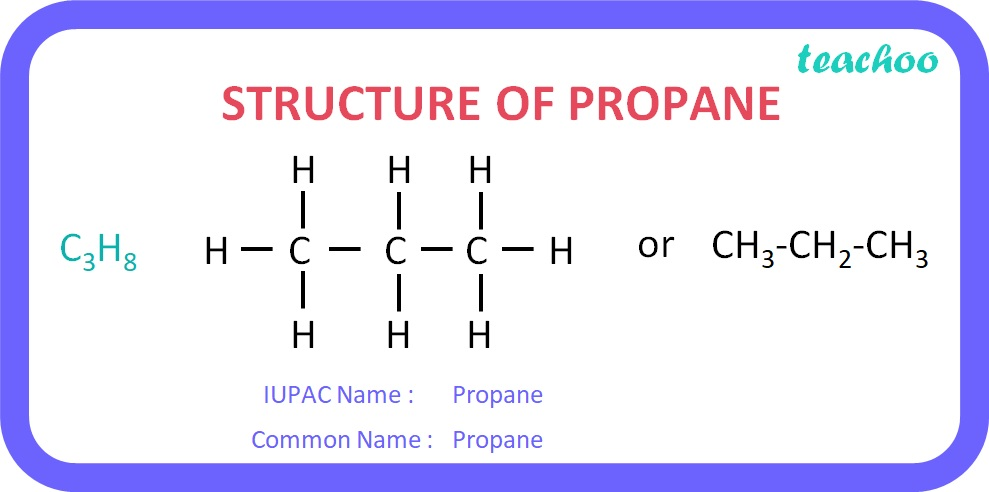Name of Alkane Formula Derivation C n H 2n+2 Number of carbon Atoms Methane CH 4 n=1 Ethane C 2 H 6 n=2 Propane C 3 H 8 n=3 Butane C 4 H 10 n=4 Pentane C 5 H 12 n=5 Hexane C 6 H 14 n=6 Heptane C 7 H 16 n=7 Octane C 8 H 18 n=8 Nonane C 9 H 20 n=9 Decane C 10 H 22 n=10

Learn in your speed, with individual attention - Teachoo Maths 1-on-1 Class